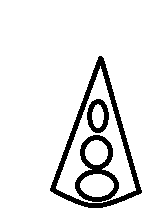# Centre of Mass

The diagram shows a pendant in the shape of a sector of a circle with center A. The radius is 4 cm and the angle at A is 0.4 radians. Three small holes of radius 0.1 cm, 0.2cm and 0.3 cm are cut away. The diameters of the holes lie along the axis of symmetry and their centers are 1, 2 and 3 cm respectively from A. The pendant can be modeled as a uniform lamina. Find the distance of the center of mass of the pendant from A.

Moments about A (y = 0 due to symmetry)

$x = \frac{(0.5\times4^2\times0.4)\times(\frac{2\times4\times(sin(0.2))}{0.6}) - (0.1^2\pi\times(1)) - (0.2^2\pi\times(2))-(0.3^2\pi\times(3))}{(0.5\times4^2\times0.4) - (0.1^2\pi) - (0.2^2\pi) - (0.3^2\pi)} => x = 2.66...$
However the answer is 2.47 :s

Last edited:

diagram?

Doc Al
Mentor
Where's the center of mass of the sector itself?The diagram was rather rubbish so I didn't include it (it's pretty much exactly like this, just the circles centers are in the line of symmetry).

I've edited my original post to include the center of mass of the sector - I just copied it wrong.